## Introduction

• Permutation tests, also called randomization tests, re-randomization tests, or exact tests
• Type of statistical significance test in which the distribution of the test statistic under the null hypothesis is obtained by calculating all possible values of the test statistic under rearrangements of the labels on the observed data points
• In other words, the method by which treatments are allocated to subjects in an experimental design is mirrored in the analysis of that design
• If the labels are exchangeable under the null hypothesis, then the resulting tests yield exact significance levels

## Permutation tests

• Significance tests tell us whether an observed effect, such as a difference between two means or a correlation between two variables, could reasonably occur just by chance in selecting a random sample
• If not, we have evidence that the effect observed in the sample reflects an effect that is present in the population
• The general construction is as follows
• Choose a statistic that measures the effect you are looking for
• Construct the sampling distribution that this statistic would have if the effect were not present in the population
• Locate the observed statistic on sampling distribution
• A value in the main body of the distribution could easily occur just by chance
• A value in the tail would rarely occur by chance and so is evidence that something other than chance is operating

Do new directed reading activities improve the reading ability of elementary school students, as measured by their Degree of Reading Power (DRP) scores? A study assigns students at random to either the new method (treatment group, 21 students) or traditional teaching methods (control group, 23 students). ${\rm statistic} = \bar x_{treatment} - \bar x_{control}$ The null hypothesis $$H_0$$ for the resampling test is that the teaching method has no effect on the distribution of DRP scores. If $$H_0$$ is true, the DRP scores do not depend on the teaching method. Each student has a DRP score that describes that child and is the same no matter which group the child is assigned to. The observed difference in group means just reflects the accident of random assignment to the two groups.

Now we can see how to resample in a way that is consistent with the null hypothesis: imitate many repetitions of the random assignment of students to treatment and control groups, with each student always keeping his or her DRP score unchanged. Because resampling in this way scrambles the assignment of students to groups, tests based on resampling are called permutation tests, from the mathematical name for scrambling a collection of things.

Permutation test procedure

1. Choose $$21$$ of the $$44$$ students at random to be the treatment group; the other 23 are the control group. This is an ordinary SRS, chosen without replacement. It is called a permutation resample. Calculate the mean DRP score in each group, using the individual DRP scores. The difference between these means is our statistic.
2. Repeat this resampling from the 44 students hundreds of times. The distribution of the statistic from these resamples estimates the sampling distribution under the condition that $$H_0$$ is true. It is called a permutation distribution.
3. Compute the actually observed value of the test statistic.
4. Find the P-value.

T <- c(24, 43, 58, 71, 61, 44, 67, 49, 59, 52, 62, 54, 46, 43, 57,
43, 57, 56, 53, 49, 33)
C <- c(42, 43, 55, 26, 33, 41, 19, 54, 46, 10, 17, 60, 37, 42, 55,
28, 62, 53, 37, 42, 20, 48, 85)
n1 <- length(T)
n2 <- length(C)
Z <- c(T,C)
N <- length(Z)
obs_stat <- mean(T)-mean(C)
B <- 1000
new_stats <- numeric(B)
for( i in 1:B){
idx <- sample(1:N,size=n1, replace=F)
newT <- Z[idx]
newC <- Z[-idx]
new_stats[i] <- mean(newT)-mean(newC)
}

pvalue <- mean(c(obs_stat,new_stats)>=obs_stat)
pvalue
##  0.00999001

hist(new_stats, main="Permutation Distribution")
points(obs_stat,0, cex=1, pch=16)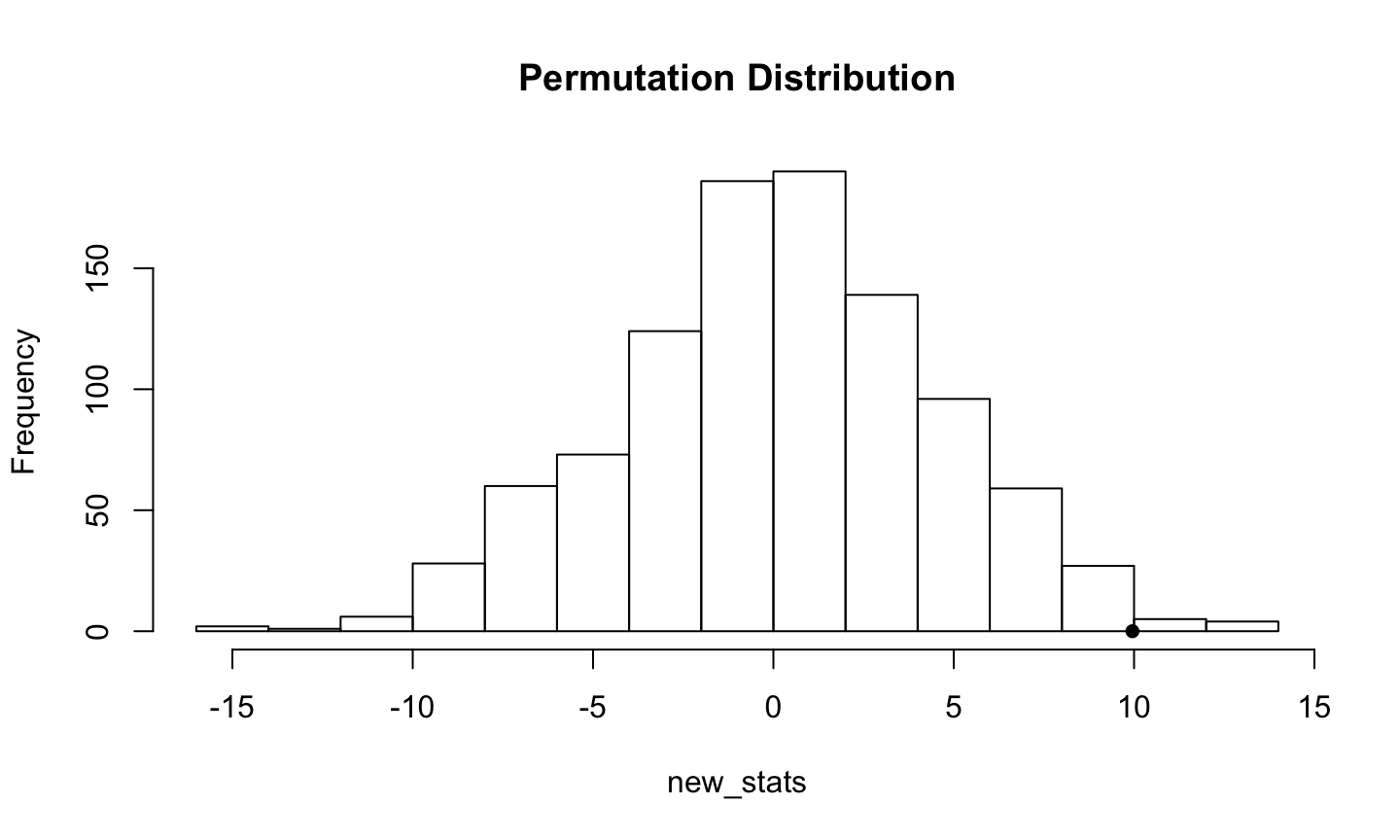## Comparison

Comparing a standard $$t$$-test approach to a permutation approach brings out some general points about permutation tests versus traditional formula-based tests

• The hypotheses for a $$t$$ test are stated in terms of population means $H_0: \mu_{treatment}-\mu_{control} =0$
• Permutation test hypotheses are more general, i.e. the null hypothesis is same distribution of scores in both groups.
• The $$t$$ test statistic is based on standardizing the difference in means in a clever way to get a statistic that has a $$t$$ distribution under $$H_0$$. The permutation test works directly with the difference of means (or some other statistic) and estimates the sampling distribution by resampling.
• The $$t$$ test gives accurate p-values when the sampling distribution of the difference of means is at least roughly normal. The permutation test gives accurate p-values even when the sampling distribution is not close to normal.

## Example: chickwts

The permutation distribution of a statistic is illustrated for a small sample, from the data set chickwts in R. Weights in grams are recorded for six groups of newly hatched chicks fed different supplements.

data(chickwts)
attach(chickwts)

## Example: chickwts

boxplot(formula(chickwts))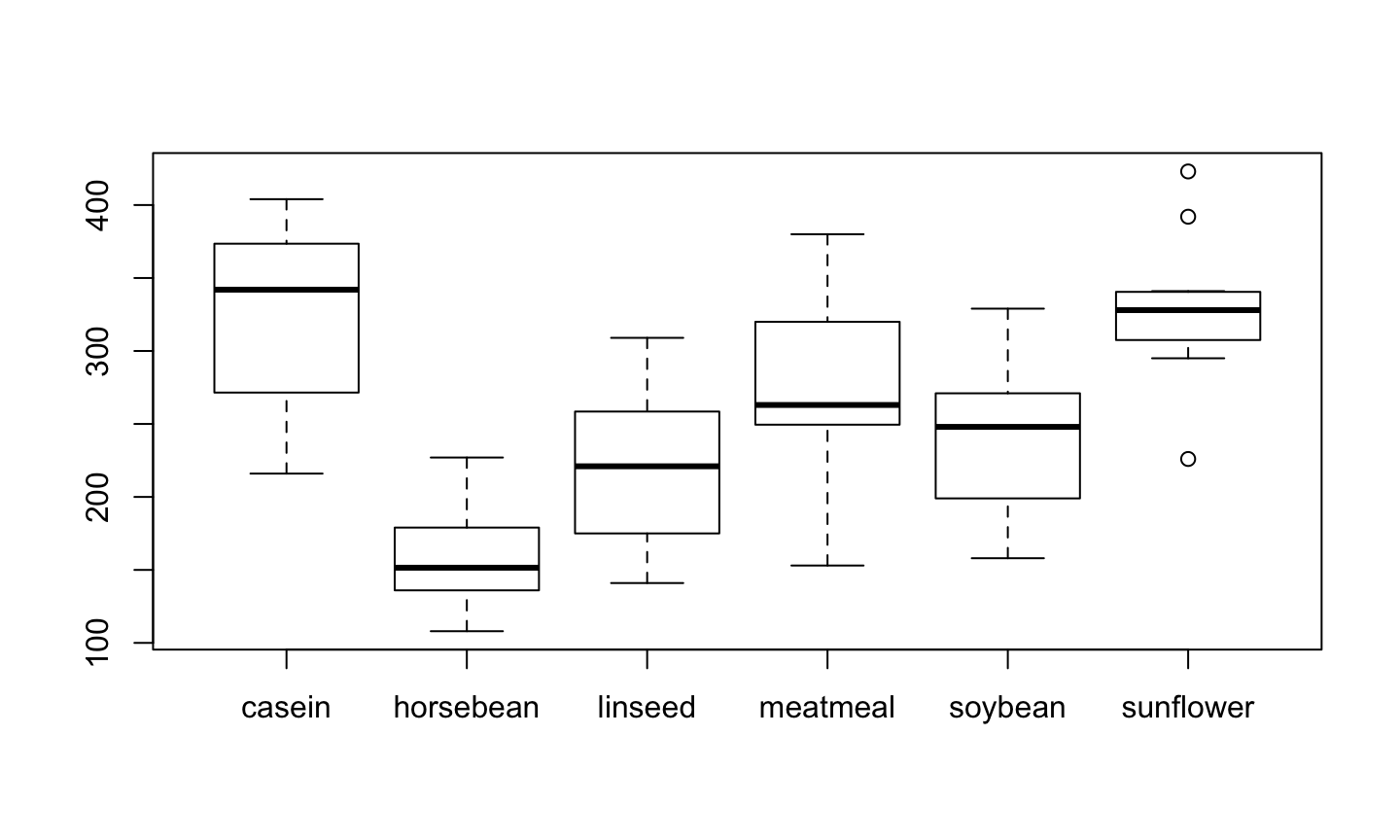## Example: chickwts

detach(chickwts)
X <- as.vector(chickwts$weight[chickwts$feed=="soybean"])
Y <- as.vector(chickwts$weight[chickwts$feed=="linseed"])

B <- 999
Z <- c(X,Y)
reps <- numeric(B)
K <- 1:26
t0 <- t.test(X,Y)$statistic for(i in 1:B){ k <- sample(K, size=14, replace=F) x1 <- Z[k] y1 <- Z[-k] reps[i] <- t.test(x1,y1)$statistic
}
p <- mean(c(t0, reps)>=t0)

## Example: chickwts

hist(reps, main="Permuation Distribution")
points(t0,0, cex=1, pch=16)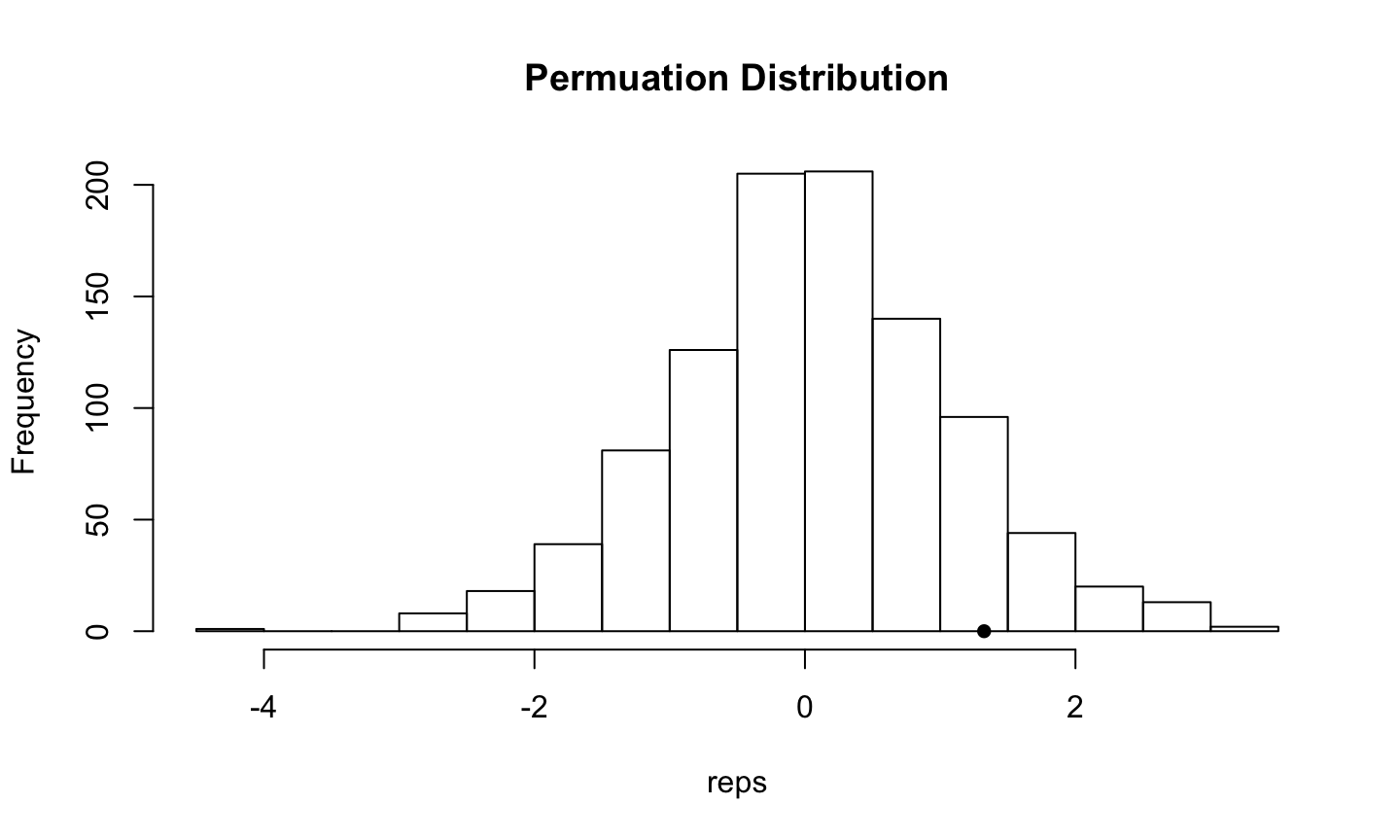## Example: K-S statistic

To apply a permutation test of equal distributions, choose a test statistic that measures the difference between two distributions, for example, the two-sample Kolmogorov-Smirnov (K-S) statistic. Consider the same example as before, but now we are interested in any type of difference in the distributions of the two groups (not just the mean value as before).

The K-S statistic $$D$$ is the maximum absolute difference between the ecdfs of the two samples, defined by $D=\sup_{1\le i\le m+n} |F_n(z_i) - G_m(z_i)|$ where $$F_n$$ is the ecdf of the first sample $$X_1, \dots, X_n$$ and $$G_m$$ is the ecdf of the second sample $$Y_1, \dots, Y_m$$. Note that $$0\le D\le 1$$ and large values of $$D$$ support the alternative $$H_1: F_X \ne F_Y$$. In R, we can compute this statistic using ks.test.

## Example: K-S statistic

D <- numeric(B)
DO <- ks.test(X,Y, exact=F)$statistic ## Warning in ks.test(X, Y, exact = F): p-value will be approximate in the ## presence of ties options(warn=-1) D <- numeric(B) for( i in 1:B){ k <- sample(K, size=14, replace=F) x1 <- Z[k] y1 <- Z[-k] D[i] <- ks.test(x1, y1, exact=F)$statistic
}
p <- mean(c(DO,D) >= DO)

## Example: K-S statistic

hist(D, main="Permuation Distribution")
points(DO,0, cex=1, pch=16)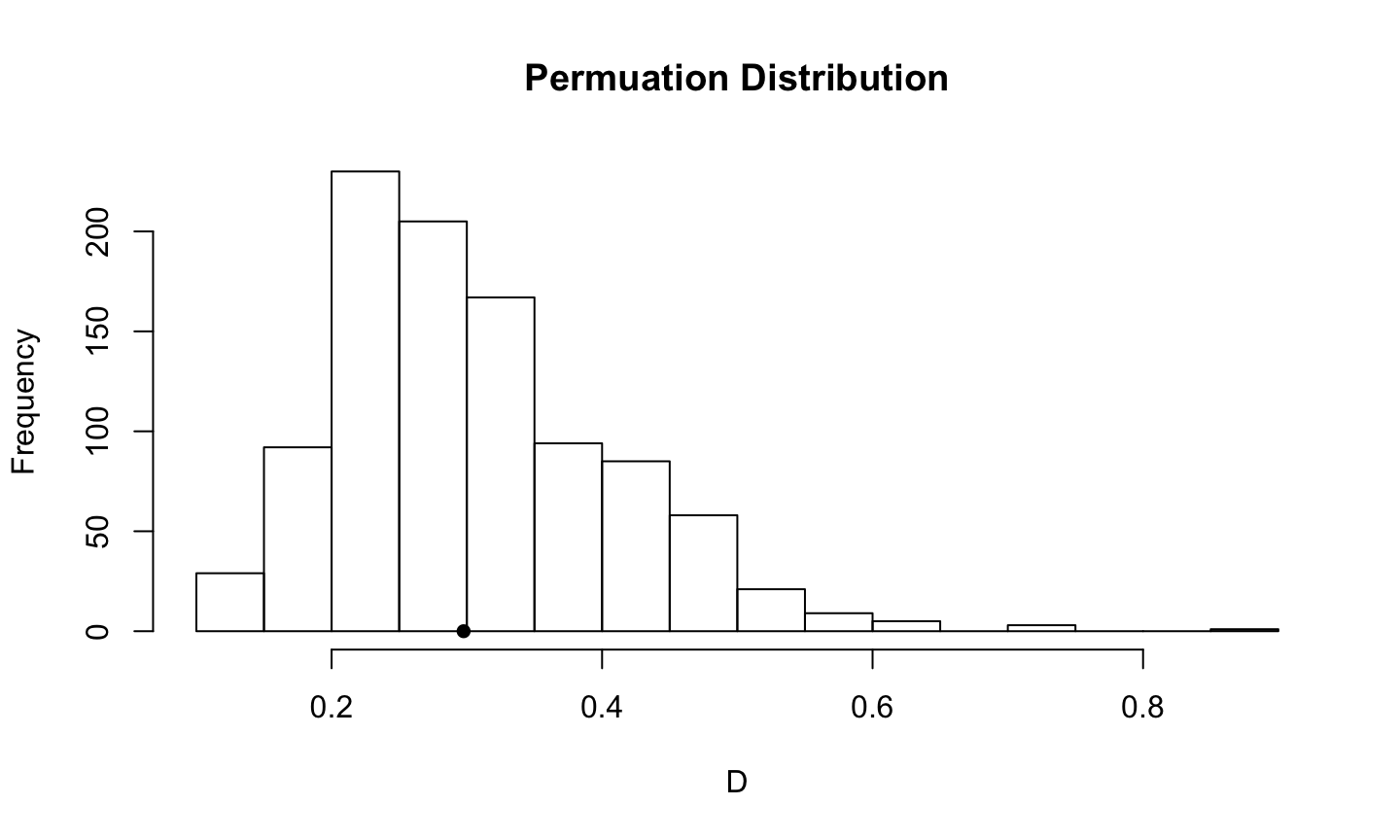## Example: Correlation coefficients

• A study by Katz et al. (1990) asked students to answer SAT-type questions without having read the passage on which those questions were based (The SAT is a standardized exam commonly used in university admissions)
• Authors looked to see how performance on such items correlated with the SAT scores those students had when they applied to college
• Expected that those students who had the skill to isolate and reject unreasonable answers, even when they couldn't know the correct answer, would also be students who would have done well on the SAT taken sometime before they came to college

## Example: Correlation coefficients

Score <- c(58,  48,  48,  41,  34,  43,  38,  53,  41,  60,  55,  44,
43, 49,  47,  33,  47,  40,  46,  53,  40,  45,  39,  47,
50,  53,  46,  53)
SAT <- c(590, 590, 580, 490, 550, 580, 550, 700, 560, 690, 800, 600,
650, 580, 660, 590, 600, 540, 610, 580, 620, 600, 560, 560,
570, 630, 510, 620)
r.obt <- cor(Score, SAT)
cat("The obtained correlation is ",r.obt,'\n')
## The obtained correlation is  0.531767

## Example: Correlation coefficients

nreps <- 5000
r.random <- numeric(nreps)
for (i in 1:nreps) {
Y <- Score
X <- sample(SAT, 28, replace = FALSE)
r.random[i] <- cor(X,Y)
}
prob <- length(r.random[r.random >= r.obt])/nreps
cat("Probability randomized r >= r.obt",prob)
## Probability randomized r >= r.obt 0.0016

## Example: Correlation coefficients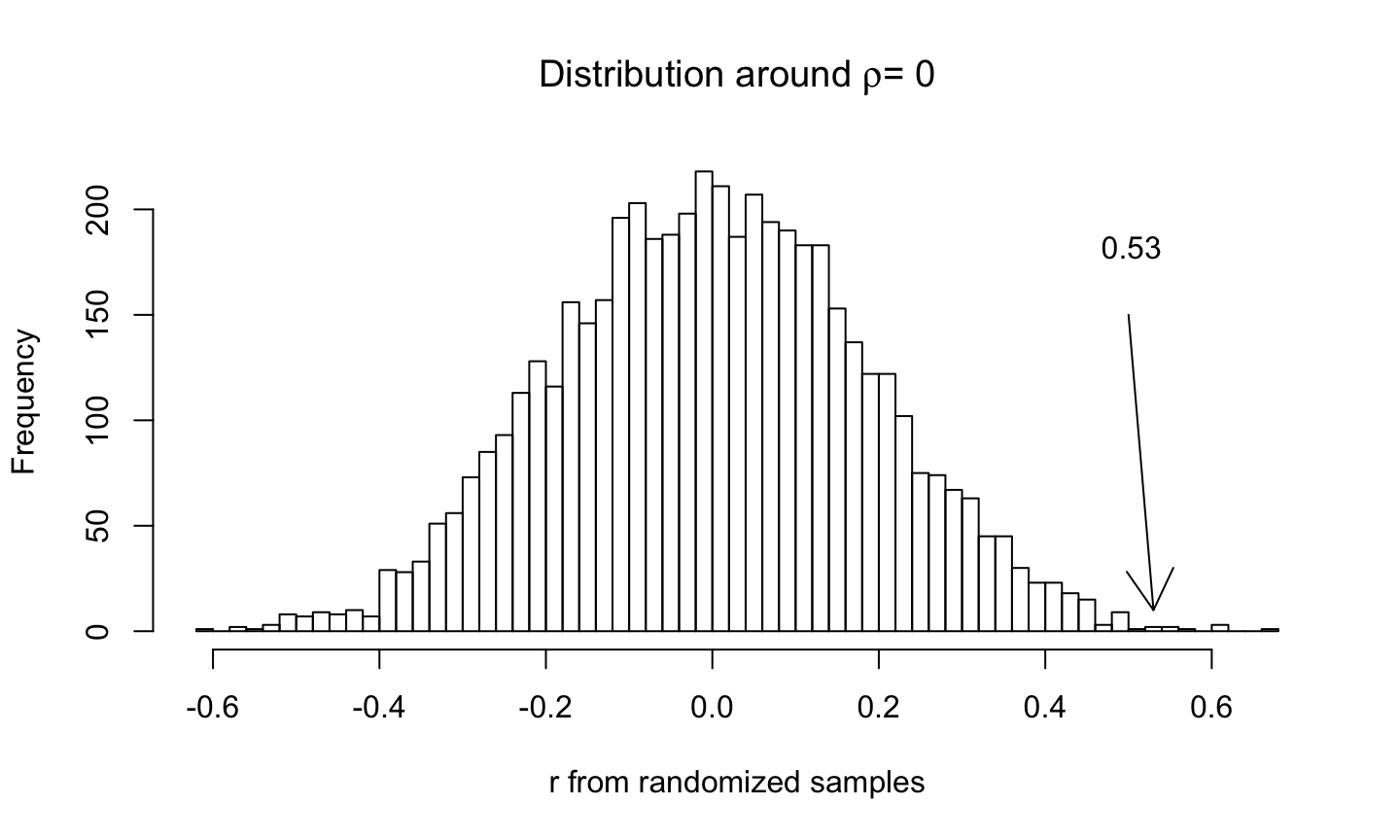## Bootstrap versus randomization

• When we bootstrap for correlations, we keep $$x_i$$ and $$y_i$$ pairs together, and randomly sample pairs of scores with replacement. That means that if one pair is 45 and 360, we will always have 45 and 360 occur together, often more than once, or neither of them will occur. What this means is that the expectation of the correlation between $$X$$ and $$Y$$ for any resampling will be the correlation in the original data.

## Bootstrap versus randomization

• When we use a randomization approach, we permute the $$Y$$ values, while holding the $$X$$ values constant. For example, if the original data were
x <- c(45, 53, 73, 80)
y <- c(22, 30, 29, 38)

then two resamples might be

rbind(x, sample(y, size=4, replace=F))
##   [,1] [,2] [,3] [,4]
## x   45   53   73   80
##     29   22   38   30
rbind(x, sample(y, size=4, replace=F))
##   [,1] [,2] [,3] [,4]
## x   45   53   73   80
##     29   22   38   30

## Bootstrap versus randomization

• Notice the top row always stays in the same order, while the bottom row is permuted randomly. This means that the expected value of the correlation between X and Y will be 0.00, not the correlation in the original sample.
• Helps to explain why bootstrapping focuses on confidence limits around $$\rho$$, whereas the randomization procedure focuses on confidence limits around 0.

## Summary

• Fisher's exact test is a commonly used permutation test for evaluating the association between two dichotomous variables
• fisher.test in R can simulate a p-value via Monte Carlo
• XNomial for larger tables
• More on Randomization Tests
• Don't always need to build our own permutation distributions, in some cases can use coin R package